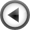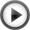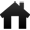# MATHEMATICS: Shortcuts in DIVISION

## Division by parts ---> imagine dividing \$874 between two persons874/2
= 800/2 + 74/2
= 400 + 37
= 437

Division using the factors of the divisor: "double division"

70/14
= (70/7)/2 ---> 7 and 2 are the factors of 14
= 10/2
= 5

Division by using fractions:

132/2
= (100/2 + 32/2) ---> break down into two fractions
= (50 + 16)
= 66

Division by 5 ---> divide by 100 then multiply by 20

1400/5
= (1400/100) x 20
= 14 x 20
= 280

Division by 10 ---> move the decimal point one place to the left

0.5/10
= 0.05 ---> 5% is 50% divided by ten

Division by 50 ---> divide by 100 then multiply by 2

2100/50
= (2100/100) x 2
= 21 x 2
= 42

700/50
= (700/100) x 2
= 7 x 2
= 14

Division by 100 ---> move the decimal point two places to the left

25/100
= 0.25

Division by 500 ---> divide by 100 then multiply by 0.2

17/500
= (17/100) x 0.2
= 0.17 x 0.2
= 0.034

Division by 25 ---> divide by 100 then multiply by 4

500/25
= (500/100) x 4
= 5 x 4
= 20

750/25
= (750/100) x 4
= 7.5 x 2 x 2
= 30

### Register Here : We Respect Our Readers' Privacy.

Subscribe Our News Letters ,Its FREE!